GFG App
Open AppBrowser
Continue

In this article, the various functions of the const keyword which is found in C++ are discussed. Whenever const keyword is attached with any method(), variable, pointer variable, and with the object of a class it prevents that specific object/method()/variable to modify its data items value.

#### Constant Variables:

There are a certain set of rules for the declaration and initialization of the constant variables:

• The const variable cannot be left un-initialized at the time of the assignment.
• It cannot be assigned value anywhere in the program.
• Explicit value needed to be provided to the constant variable at the time of declaration of the constant variable.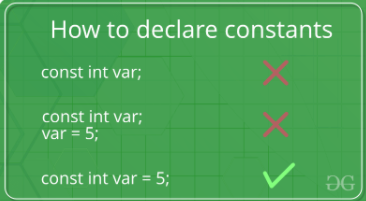Below is the C++ program to demonstrate the above concept:

## C++

 `// C++ program to demonstrate the` `// the above concept` `#include ` `using` `namespace` `std;`   `// Driver Code` `int` `main()` `{`   `    ``// const int x;  CTE error` `    ``// x = 9;   CTE error` `    ``const` `int` `y = 10;` `    ``cout << y;`   `    ``return` `0;` `}`

Output:

`10`

The error faced for faulty declaration: If you try to initialize the const variable without assigning an explicit value then a compile-time error (CTE) is generated.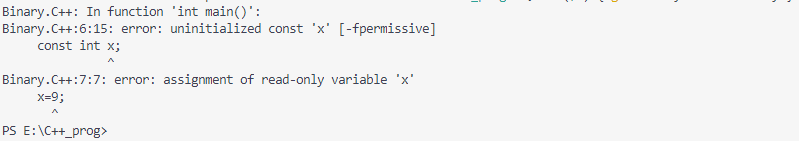### Const Keyword With Pointer Variables:

Pointers can be declared with a const keyword. So, there are three possible ways to use a const keyword with a pointer, which are as follows:

When the pointer variable point to a const value:

Syntax:

`const data_type* var_name;`

Below is the C++ program to implement the above concept:

## C++

 `// C++ program to demonstrate the` `// above concept` `#include ` `using` `namespace` `std;`   `// Driver Code` `int` `main()` `{` `    ``int` `x{ 10 };` `    ``char` `y{ ``'M'` `};`   `    ``const` `int``* i = &x;` `    ``const` `char``* j = &y;`   `    ``// Value of x and y can be altered,` `    ``// they are not constant variables` `    ``x = 9;` `    ``y = ``'A'``;`   `    ``// Change of constant values because,` `    ``// i and j are pointing to const-int` `    ``// & const-char type value` `    ``// *i = 6;` `    ``// *j = 7;`   `    ``cout << *i << ``" "` `<< *j;` `}`

Output:

`9 A`

Explanation: Here in the above case, i and j are two pointer variables that are pointing to a memory location const int-type and char-type, but the value stored at these corresponding locations can be changed as we have done above.

Otherwise, the following error will appear: If we try to modify the value of the const variable.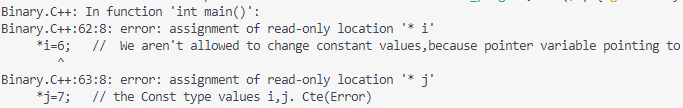When the const pointer variable point to the value:

Syntax:

`data_type* const var_name;`

Below is the example to demonstrate the above concept:

## C++

 `// C++ program to demonstrate the ` `// above concept ` `#include ` `using` `namespace` `std; `   `// Driver Code ` `int` `main() ` `{ ` `    ``// x and z non-const var ` `    ``int` `x = 5; ` `    ``int` `z = 6; `   `    ``// y and p non-const var ` `    ``char` `y = ``'A'``; ` `    ``char` `p = ``'C'``; `   `    ``// const pointer(i) pointing ` `    ``// to the var x's location ` `    ``int``* ``const` `i = &x; `   `    ``// const pointer(j) pointing ` `    ``// to the var y's location ` `    ``char``* ``const` `j = &y; `     `    ``// The values that is stored at the memory location can modified ` `    ``// even if we modify it through the pointer itself` `    ``// No CTE error` `    ``*i = 10;` `    ``*j = ``'D'``;`   `    ``// CTE because pointer variable ` `    ``// is const type so the address ` `    ``// pointed by the pointer variables ` `    ``// can't be changed ` `    ``// i = &z; ` `    ``// j = &p; `   `    ``cout << *i << ``" and "` `<< *j ` `        ``<< endl; ` `    ``cout << i << ``" and "` `<< j; `   `    ``return` `0; ` `} `

```Output:
10 and D
0x7ffe7b2392a0 and DC```

Explanation: The values that are stored in the corresponding pointer variable i and j are modifiable, but the locations that are pointed out by const-pointer variables where the corresponding values of x and y are stored aren’t modifiable.

Otherwise, the following error will appear: The pointer variables are const and pointing to the locations where the x and y are stored if we try to change the address location then we’ll face the error.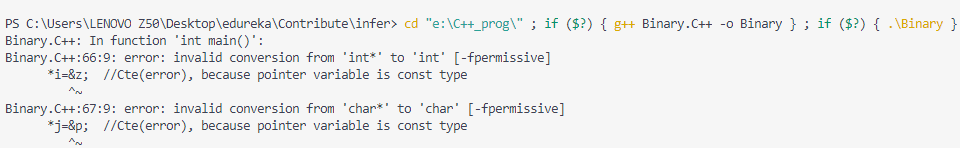When const pointer pointing to a const variable:

Syntax:

`const data_type* const var_name;`

Below is the C++ program to demonstrate the above concept:

## C++

 `// C++ program to demonstrate` `// the above concept` `#include ` `using` `namespace` `std;`   `// Driver code` `int` `main()` `{` `    ``int` `x{ 9 };`   `    ``const` `int``* ``const` `i = &x;` `  `  `    ``// *i=10;   ` `    ``// The above statement will give CTE` `    ``// Once Ptr(*i) value is` `    ``// assigned, later it can't` `    ``// be modified(Error)`   `    ``char` `y{ ``'A'` `};`   `    ``const` `char``* ``const` `j = &y;` `  `  `    ``// *j='B'; ` `    ``// The above statement will give CTE` `    ``// Once Ptr(*j) value is` `    ``// assigned, later it can't` `    ``// be modified(Error)`   `    ``cout << *i << ``" and "` `<< *j;`   `    ``return` `0;` `}`

Output:

`9 and A`

Explanation: Here, the const pointer variable points to the const variable. So, you are neither allowed to change the const pointer variable(*P) nor the value stored at the location pointed by that pointer variable(*P).

Otherwise, the following error will appear: Here both pointer variable and the locations pointed by the pointer variable are const so if any of them is modified, the following error will appear: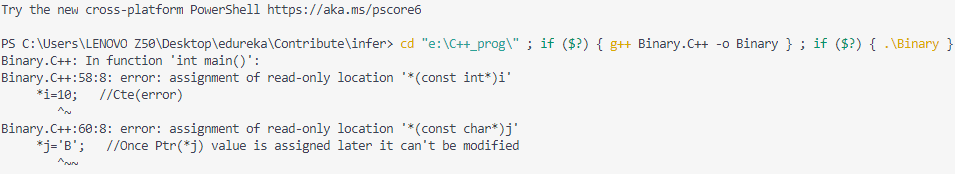Pass const-argument value to a non-const parameter of a function cause error: Passing const argument value to a non-const parameter of a function isn’t valid it gives you a compile-time error.

Below is the C++ program to demonstrate the above concept:

## C++

 `// C++ program to demonstrate` `// the above concept` `#include ` `using` `namespace` `std;`   `int` `foo(``int``* y)` `{` `    ``return` `*y;` `}`   `// Driver code` `int` `main()` `{` `    ``int` `z = 8;` `    ``const` `int``* x = &z;` `    ``cout << foo(x);` `    ``return` `0;` `}`

Output: The compile-time error that will appear as if const value is passed to any non-const argument of the function then the following compile-time error will appear: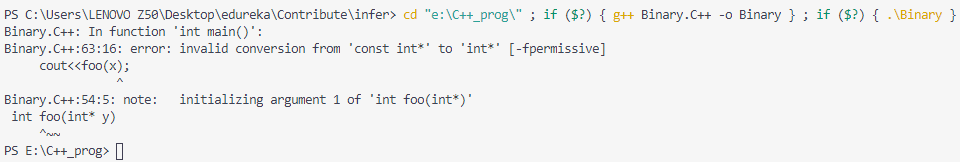In nutshell, the above discussion can be concluded as follows:

1. int value = 5;         // non-const value

2. const int *ptr_1 = &value;      // ptr_1 points to a “const int” value, so this is a pointer to a const value.

3. int *const ptr_2 = &value;        // ptr_2 points to an “int”, so this is a const pointer to a non-const value.

4. const int *const ptr_3 = &value;   // ptr_3 points to a “const int” value, so this is a const pointer to a const value.

### Constant Methods:

Like member functions and member function arguments, the objects of a class can also be declared as const. An object declared as const cannot be modified and hence, can invoke only const member functions as these functions ensure not to modify the object.

Syntax:

`const Class_Name Object_name;`
• When a function is declared as const, it can be called on any type of object, const object as well as non-const objects.
• Whenever an object is declared as const, it needs to be initialized at the time of declaration. However, the object initialization while declaring is possible only with the help of constructors.

There are two ways of a constant function declaration:

Ordinary const-function Declaration:

```const void foo()
{
//void foo() const Not valid
}
int main()
{
foo();
}  ```

A const member function of the class:

```class
{
void foo() const
{
//.....
}
}```

Below is the example of a constant function:

## C++

 `// C++ program to demonstrate the` `// constant function` `#include ` `using` `namespace` `std;`   `// Class Test` `class` `Test {` `    ``int` `value;`   `public``:` `    ``// Constructor` `    ``Test(``int` `v = 0)` `    ``{` `        ``value = v;` `    ``}`   `    ``// We get compiler error if we` `    ``// add a line like "value = 100;"` `    ``// in this function.` `    ``int` `getValue() ``const` `    ``{` `        ``return` `value;` `    ``}` `    `  `    ``// a nonconst function trying to modify value` `    ``void` `setValue(``int` `val) {` `        ``value = val;` `    ``}` `};`   `// Driver Code` `int` `main()` `{` `    ``// Object of the class T` `    ``Test t(20);`   `    ``// non-const object invoking const function, no error` `    ``cout << t.getValue() << endl;` `    `  `    ``// const object` `      ``const` `Test t_const(10);` `  `  `    ``// const object invoking const function, no error` `    ``cout << t_const.getValue() << endl;` `  `  `    ``// const object invoking non-const function, CTE` `    ``// t_const.setValue(15);` `    `  `    ``// non-const object invoking non-const function, no error` `    ``t.setValue(12);` `    `  `    ``cout << t.getValue() << endl;`   `    ``return` `0;` `}`

Output:

`20`

The Following error will if you try call the non-const function from a const object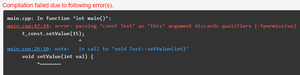### Constant Function Parameters And Return Type:

A function() parameters and return type of function() can be declared as constant. Constant values cannot be changed as any such attempt will generate a compile-time error.

Below is the C++ program to implement the above approach:

## C++

 `// C++ program to demonstrate the` `// above approach` `#include ` `using` `namespace` `std;`   `// Function foo() with variable` `// const int` `void` `foo(``const` `int` `y)` `{` `    ``// y = 6; const value` `    ``// can't be change` `    ``cout << y;` `}`   `// Function foo() with variable int` `void` `foo1(``int` `y)` `{` `    ``// Non-const value can be change` `    ``y = 5;` `    ``cout << ``'\n'` `         ``<< y;` `}`   `// Driver Code` `int` `main()` `{` `    ``int` `x = 9;` `    ``const` `int` `z = 10;` `  `  `    ``foo(z);` `    ``foo1(x);`   `    ``return` `0;` `}`

Output:

```10
5```

Explanation: The following error will be displayed:

• // y = 6; a const value can’t be changed or modified.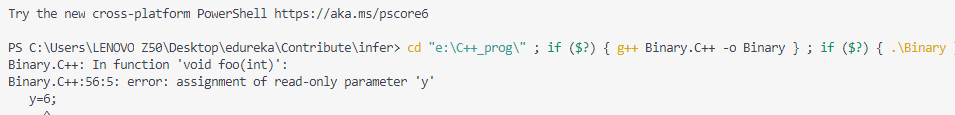For const return type: The return type of the function() is const and so it returns a const integer value to us. Below is the C++ program to implement the above approach:

## C++

 `// C++ program for the above approach` `#include ` `using` `namespace` `std;`   `const` `int` `foo(``int` `y)` `{` `    ``y--;` `    ``return` `y;` `}`   `int` `main()` `{` `    ``int` `x = 9;` `    ``const` `int` `z = 10;` `    ``cout << foo(x) << ``'\n'` `         ``<< foo(z);`   `    ``return` `0;` `}`

Output:

```8
9```

There is no substantial issue to pass const or non-const variable to the function because the value that will be returned by the function will be constant automatically. As the argument of the function is non-const.

For const return type and const parameter: Here, both return type and parameter of the function are of const types. Below is the C++ program to implement the above approach:

## C++

 `// C++ program for the above approach` `#include ` `using` `namespace` `std;`   `const` `int` `foo(``const` `int` `y)` `{` `    ``// y = 9; it'll give CTE error as` `    ``// y is const var its value can't` `    ``// be change` `    ``return` `y;` `}`   `// Driver code` `int` `main()` `{` `    ``int` `x = 9;` `    ``const` `int` `z = 10;` `    ``cout << foo(x) << ``'\n'` `         ``<< foo(z);`   `    ``return` `0;` `}`

Output:

```9
10```

Explanation: Here, both const and non-const values can be passed as the const parameter to the function, but we are not allowed to then change the value of a passed variable because the parameter is const. Otherwise, we’ll face the error as below:

// y=9; it’ll give the compile-time error as y is const var its value can’t be changed.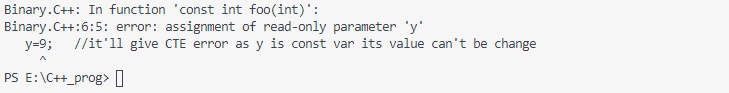My Personal Notes arrow_drop_up